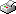Print This Page Jessica Franzén -- doctoral thesis -- Bayesian Cluster Analysis - Some Extensions to Non-standard Situations Abstract The Bayesian approach to cluster analysis is presented. We assume that all data stem from a finite mixture model, where each component corresponds to one cluster and is given by a multivariate normal distribution with unknown mean and variance. The method produces posterior distributions of all cluster parameters and proportions as well as associated cluster probabilities for all objects. We extend this method in several directions to some common but non-standard situations. The first extension covers the case with a few deviant observations not belonging to one of the normal clusters. An extra component/cluster is created for them, which has a larger variance or a different distribution, e.g. is uniform over the whole range. The second extension is clustering of longitudinal data. All units are clustered at all time points separately and the movements between time points are modeled by Markov transition matrices. This means that the clustering at one time point will be affected by what happens at the neighbouring time points. The third extension handles datasets with missing data, e.g. item non-response. We impute the missing values iteratively in an extra step of the Gibbs sampler estimation algorithm. The Bayesian inference of mixture models has many advantages over the classical approach. However, it is not without computational difficulties. A software package, written in Matlab for Bayesian inference of mixture models, is introduced. The programs of the package handle the basic cases of clustering data that are assumed to arise from mixture models of multivariate normal distributions, as well as the non-standard situations. Keywords: Cluster analysis, Clustering, Classification, Mixture model, Gaussian, Bayesian inference, MCMC, Gibbs sampler, Deviant group, Longitudinal, Missing data, Multiple imputation. ISBN 978-91-7155-645-5